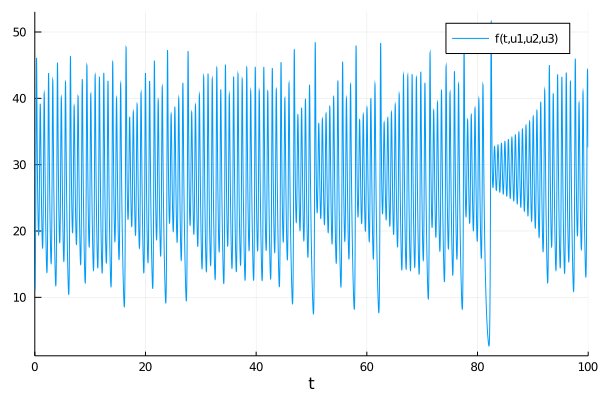# Plot Functions

## Standard Plots Using the Plot Recipe

Plotting functionality is provided by recipes to Plots.jl. To plot solutions, simply call the plot(type) after importing Plots.jl and the plotter will generate appropriate plots.

#]add Plots # You need to install Plots.jl before your first time using it!
using Plots
plot(sol) # Plots the solution

Many of the types defined in the DiffEq universe, such as ODESolution, ConvergenceSimulation WorkPrecision, etc. have plot recipes to handle the default plotting behavior. Plots can be customized using all of the keyword arguments provided by Plots.jl. For example, we can change the plotting backend to the GR package and put a title on the plot by doing:

gr()
plot(sol,title="I Love DiffEqs!")

Then to save the plot, use savefig, for example:

savefig("myplot.png")

## Density

If the problem was solved with dense=true, then denseplot controls whether to use the dense function for generating the plot, and plotdensity is the number of evenly-spaced points (in time) to plot. For example:

plot(sol,denseplot=false)

means "only plot the points which the solver stepped to", while:

plot(sol,plotdensity=1000)

means to plot 1000 points using the dense function (since denseplot=true by default).

## Choosing Variables

In the plot command, one can choose the variables to be plotted in each plot. The master form is:

vars = [(f1,0,1), (f2,1,3), (f3,4,5)]

which could be used to plot f1(var₀, var₁), f2(var₁, var₃), and f3(var₄, var₅), all on the same graph. (0 is considered to be time, or the independent variable). Functions f1, f2 and f3 should take in scalars and return a tuple. If no function is given, for example,

vars = [(0,1), (1,3), (4,5)]

this would mean "plot var₁(t) vs t (time), var₃(var₁) vs var₁, and var₅(var₄) vs var₄ all on the same graph, putting the independent variables (t, var₁ and var₄) on the x-axis." While this can be used for everything, the following conveniences are provided:

• Everywhere in a tuple position where we only find an integer, this variable is plotted as a function of time. For example, the list above is equivalent to:
vars = [1, (1,3), (4,5)]

and

vars = [1, 3, 4]

is the most concise way to plot the variables 1, 3, and 4 as a function of time.

• It is possible to omit the list if only one plot is wanted: (2,3) and 4 are respectively equivalent to [(2,3)] and [(0,4)].

• A tuple containing one or several lists will be expanded by associating corresponding elements of the lists with each other:

vars = ([1,2,3], [4,5,6])

is equivalent to

vars = [(1,4), (2,5), (3,6)]

and

vars = (1, [2,3,4])

is equivalent to

vars = [(1,2), (1,3), (1,4)]
• Instead of using integers, one can use the symbols from a ParameterizedFunction. For example, vars=(:x,:y) will replace the symbols with the integer values for components :x and :y.

• n-dimensional groupings are allowed. For example, (1,2,3,4,5) would be a 5-dimensional plot between the associated variables.

### Complex Numbers and High Dimensional Plots

The recipe library DimensionalPlotRecipes.jl is provided for extra functionality on high dimensional numbers (complex numbers) and other high dimensional plots. See the README for more details on the extra controls that exist.

### Timespan

A plotting timespan can be chosen by the tspan argument in plot. For example:

plot(sol,tspan=(0.0,40.0))

only plots between t=0.0 and t=40.0. If denseplot=true these bounds will be respected exactly. Otherwise the first point inside and last point inside the interval will be plotted, i.e. no points outside the interval will be plotted.

### Example

using DifferentialEquations, Plots
function lorenz(du,u,p,t)
du = p*(u-u)
du = u*(p-u) - u
du = u*u - p*u
end

u0 = [1., 5., 10.]
tspan = (0., 100.)
p = (10.0,28.0,8/3)
prob = ODEProblem(lorenz, u0, tspan,p)
sol = solve(prob)
xyzt = plot(sol, plotdensity=10000,lw=1.5)
xy = plot(sol, plotdensity=10000, vars=(1,2))
xz = plot(sol, plotdensity=10000, vars=(1,3))
yz = plot(sol, plotdensity=10000, vars=(2,3))
xyz = plot(sol, plotdensity=10000, vars=(1,2,3))
plot(plot(xyzt,xyz),plot(xy, xz, yz, layout=(1,3),w=1), layout=(2,1))An example using the functions:

f(x,y,z) = (sqrt(x^2+y^2+z^2),x)
plot(sol,vars=(f,1,2,3))or the norm over time:

f(t,x,y,z) = (t,sqrt(x^2+y^2+z^2))
plot(sol,vars=(f,0,1,2,3))## Animations

Using the iterator interface over the solutions, animations can also be generated via the animate(sol) command. One can choose the filename to save to via animate(sol,filename), while the frames per second fps and the density of steps to show every can be specified via keyword arguments. The rest of the arguments will be directly passed to the plot recipe to be handled as normal. For example, we can animate our solution with a larger line-width which saves every 4th frame via:

#]add ImageMagick # You may need to install ImageMagick.jl before your first time using it!
#using ImageMagick # Some installations require using ImageMagick for good animations
animate(sol,lw=3,every=4)

## Plotting Without the Plot Recipe

What if you don't want to use Plots.jl? Odd choice, but that's okay! If the differential equation was described by a vector of values, then the solution object acts as an AbstractMatrix sol[i,j] for the ith variable at timepoint j. You can use this to plot solutions. For example, in PyPlot, Gadfly, GR, etc., you can do the following to plot the timeseries:

plot(sol.t,sol')

since these plot along the columns, and sol' has the timeseries along the column. Phase plots can be done similarly, for example:

plot(sol[i,:],sol[j,:],sol[k,:])

is a 3d phase plot between variables i, j, and k.

Notice that this does not use the interpolation. When not using the plot recipe, the interpolation must be done manually. For example:

n = 101 #number of timepoints
ts = range(0, stop=1, length=n)
plot(sol(ts,idxs=i),sol(ts,idxs=j),sol(ts,idxs=k))

is the phase space using values 0.01 apart in time.